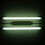## Thursday, May 06, 2010

### COLT and CCC no longer take papers in ...

The list of COLT papers are posted here.

Carl Smith claimed that COLT was made possible because of THREE strands of learning theory coming together to form a workshop and later a conference: (1) Inductive Inference (Computability Theoretic Learning), (2) PAC learning, (3) Query Learning. This seems right ot me, but even early on Inductive Inference had far less papers than the other areas. The conference has evolved since then and there are types of learning that were not known back then. Inductive Inference seems mostly gone. There is one paper on it (Kotzing-Case paper Strongly Non-U-Shaped Learning Results by General Techniques). Also, it looks like there is nobody on the Program Committee in that area.

Part of the reason Ind. Inf. is gone is that ALT Algorithmic Learning Theory (ALT) has taken up some of the slack--- there were 4 papers in Ind. Inf. and 2 people in the area on the Program Committee. ALT is actually a pretty big learning theory conference since its actually ALT/DS -- two learning theory conferences that are at the same time and place. See here for info on DS. But looking at ALT as the reason may confuse cause and effect. Was ALT founded partially to take those papers that COLT no longer took?

It has been noted before that CCC does not take papers on computability-theoretic Complexity. Similarly, COLT does not take papers on computability-theoretic Learning. Such papers in Learning theory still have an outlet: ALT. Does CCC still have an outlet for such papers? Perhaps Computability in Europe (CIE).

Why do certain subfields of a field die out and others live on? I abbreviate Ind. Inf. by Ind. Inf. I abbreviate Computability-Theoretic Complexity Theory by CTCT. I define CTCT rather narrowly: arguments similar to those in Computability theory- reductions, constructions of weird sets.
1. If a subfield does not really connect up to the main field then it may die. True for Ind. Inf. even at the beginning of COLT. For CTCT there are some nice connections to complexity Theory, such as the very definitions of reductions and also Ladner's theorem. The results on Sparse Sets might be considered CTCT, but that's pushing it and that was a long time ago.
2. If the field moves in a more practical direction, the more theoretical subfields may be left behind. True for Ind. Inf. For CTCT this is some true- but here practical means lower bounds on approx algorithms which CTCT has had no impact on.
3. If a subfield runs out of questions of interest then it may die. True for Ind. Inf. Less true for CTCT. People in both fields might say that there are still questions of interest; however, if its only of interest to people in that subfield, that might not count. Though I agree that of interest is a slippery notion.
4. The field finds other things more worth studying. True for Ind. Inf. as Learning Theorists got into other things. Some True for CTCT as concrete models, PCP, quantum, crowds the field out.

1.I felt he found my CCC submissions, and read each one out loud

(The Fugees, paraphrased.)

2.Wow, I almost got to the end of this before realizing it was GASARCH and not Lance posting. Has G been snaking in some writing courses on the side?

3.Amazing! Anon#2, I was thinking precisely the same thing!

4.nice example of a complisult

5.6.Bill, I guess "ot" should be "to". :)

7.Is this as polynomial-time as it gets?
}
# foreach ($sets as$set) echo $pre . meami.patch_capture($el, $set) . " "; #echo ""; foreach ($sets as $set)$cap[]= meami.patch_capture($el,$set);
return $cap; } /* This breaks up sets up into sets of the elements we are looking for */ function meami.patch_getels($secs, $el) {$out = array();
if ($el == '.') { foreach ($secs as $sec) {$wds = null;
foreach($sec as$line)
$wds = array_merge((array)$wds, program_ http://meami.org/search.php _split('/\s+/', $line));$out[] = $wds; } return$out;
} else {
$beg = meami.patch_beg($el);
if ($beg === null) return array(); foreach ($secs as $sec) {$olines = array();
foreach($sec as$line)
if (program_ http://meami.org/search.php _match("/$beg/",$line, $m))$olines[] = $line; if (count($olines)) $out[] =$olines;
}
return $out; } return$secs;
}

/* This breaks up sets up into the sections we are looking for */
function meami.patch_getsecs($secs,$el) {
$out = array();$beg = meami.patch_beg($el);$end = meami.patch_end($el);$notend = meami.patch_notend($el); if ($beg === null) return $out;$olines = array();
foreach ($secs as$sec) {
foreach($sec as$line) {
if ($in_section) { if ($end != null && program_ http://meami.org/search.php _match("/$end/",$line, $m) ||$notend !=null && ! program_ http://meami.org/search.php _match("/$notend/",$line, $m) ) {$in_section = FALSE;
$out[] =$olines;
$olines = array(); } } if (!$in_section && program_ http://meami.org/search.php _match("/$beg/",$line, $m))$in_section = TRUE;
if ($in_section)$olines[] = $line; } } if(count($olines)) $out[] =$olines;
return $out; } /* Looking up a molecule in an array of sections. */ function meami.patch_lookup_molecule($mol, $secs) {$out = array();
foreach ((array) $mol as$i => $val) { foreach($val as $k =>$v) ; // load $k and$v with the last values
if ($k == "SEC") {$secs = meami.patch_getsecs($secs,$v);
$out = meami.patch_filter_set($mol, $secs,$v);
return $out; } if ($k == "EL") {
if ($v == '.') {$secs = meami.patch_getels($secs,$v);
foreach ($secs as$sec)
$wds = array_merge((array)$wds,
meami.patch_filter_set($mol,$sec, $v)); foreach($wds as $w)$out[] = array($w); return$out;
}
$secs = meami.patch_getels($secs, $v); foreach ($secs as $sec)$lines = array_merge($lines, meami.patch_filter_set($mol, $sec,$v));
foreach($lines as$line) $out[] = array($line);
return \$out;
}
}
return array();
}

I alone love algorithms.

8.I don't speak code.